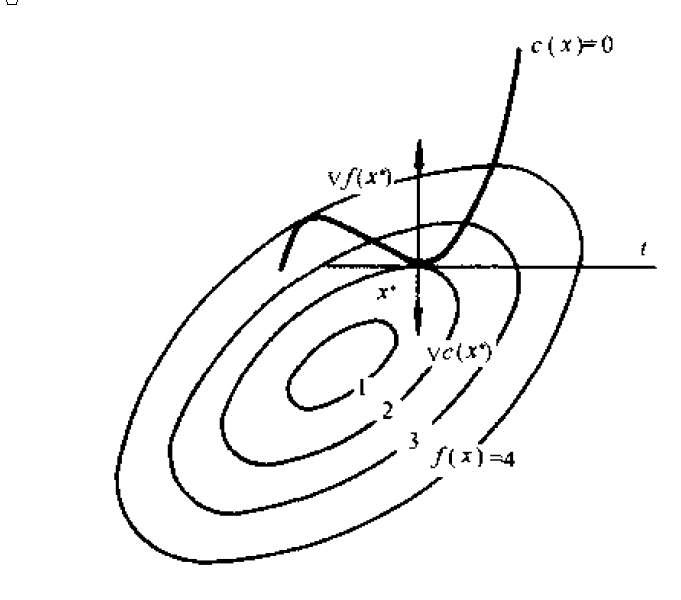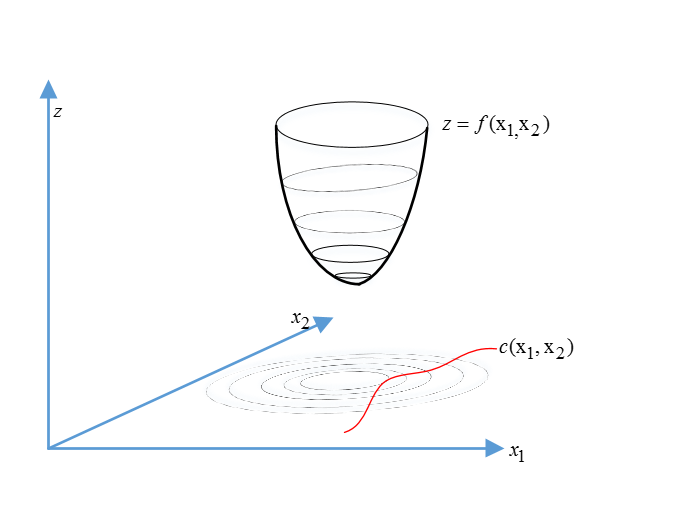# KKT条件和拉格朗日乘子法

hit2015spring $\frac{hit2015spring}{晨凫追风}$，转载请注明出处

## 无约束问题的解的必要条件

f(x) $f(x)$ x $x$处的梯度向量是0

## 有约束问题的最优性条件

### 等式约束问题的必要条件：

#### 一个条件，两变量

minf(x)=f([x]1,[x]2)$min f(x)=f([x]_1,[x]_2)$
s.t.c(x)=c([x]1,[x]2)=0 $s.t. c(x)=c([x]_1,[x]_2)=0$

f(x)+αc(x)=0 $\triangledown f(x^*)+\alpha^* \triangledown c(x^*)=0$
c(x)=0 $c(x^*)=0$

α $\alpha^*$是常数，即存在一个常数使得约束条件和待优化问题的梯度之和为0

xc(x1,x2)=0 $x满足c(x_1,x_2)=0$的条件下去寻找一个 x $\mathbf x$来使得 f(x) $f(x)$最小。其实这个点就是在满足约束条件 c(x)=0 $c(x)=0$的曲面上。c(x) $c(x)$是曲线，画出 f(x)=k $f(x)=k$的等高线，当然了在 x=4 $x=4$的位置两者相交，但是二者不是相切的，这样就还能移动x点去寻找更低的点来满足最小化，其实这里要找的点就是二者相切的点，这样就能保证最小值，相切的时候，目标函数的梯度肯定正交于约束曲面，此时就有了
f(x)+αc(x)=0 $\triangledown f(x^*)+\alpha^* \triangledown c(x^*)=0$f(x)+αc(x)=0 $\triangledown f(x^*)+\alpha^* \triangledown c(x^*)=0$

L(x,α)=L([x]1,[x]2,α)=f([x]1,[x]2)+αc([x]1,[x]2) $L(x,\alpha)=L([x]_1,[x]_2,\alpha)=f([x]_1,[x]_2)+\alpha c([x]_1,[x]_2)$

xL(x,α)=f(x)+αc(x)=0 $\triangledown_x L(x^*,\alpha^*)=\triangledown f(x^*)+\alpha^* \triangledown c(x^*)=0$
αL(x,α)=c(x)=0 $\triangledown_\alpha L(x^*,\alpha^*)=c(x^*)=0$

#### 推广到两个约束条件，三个变量的问题

minf(x)=f([x]1,[x]2,[x]3) $min f(x)=f([x]_1,[x]_2,[x]_3)$
s.t.ci(x)=ci([x]1,[x]2,[x]3)=0 $s.t. c_i(x)=c_i([x]_1,[x]_2,[x]_3)=0$

f(x)+α1c1(x)+α2c2(x)=0 $\triangledown f(x^*)+\alpha^*_1 \triangledown c_1(x^*)+\alpha^*_2 \triangledown c_2(x^*)=0$
c1(x)=0 $c_1(x^*)=0$
c2(x)=0 $c_2(x^*)=0$

L(x,α)=L([x]1,[x]2,[x]3,α1,α2)=f([x]1,[x]2[x]3)+α1c1([x]1,[x]2[x]3)+α2c2([x]1,[x]2[x]3) $L(x,\alpha)=L([x]_1,[x]_2,[x]_3,\alpha_1,\alpha_2)=f([x]_1,[x]_2，[x]_3)+\alpha_1 c_1([x]_1,[x]_2，[x]_3)+\alpha_2 c_2([x]_1,[x]_2，[x]_3)$

xL(x,α)=f(x)+α1c1(x)+α2c2(x)=0 $\triangledown_x L(x^*,\alpha^*)=\triangledown f(x^*)+\alpha^*_1 \triangledown c_1(x^*)+\alpha^*_2 \triangledown c_2(x^*)=0$
αL(x,α)=c(x)=0 $\triangledown_\alpha L(x^*,\alpha^*)=\mathbf c(x^*)=0$

L(x,α)=L([x]1,[x]2,,[x]n,α1,,αq)=f([x]1,[x]2)+i=1qαici([x]1,[x]2)=f(x)+αTc(x)$L(x,\alpha)=L([x]_1,[x]_2,\cdots,[x]_n,\alpha_1,\cdots,\alpha_q)=f([x]_1,[x]_2)+\sum\limits_{i=1}^q\alpha_i c_i([x]_1,[x]_2)=f(x)+\alpha^Tc(x)$

x=([x]1,[x]2,,[x]n) $x^* = ([x]_1^*,[x]_2^*,\cdots,[x]_n^*)$是该问题的局部最优解，且约束函数在 x $x^*$处的梯度向量 c1(x),,cq(x) $\triangledown c_1(x^*),\cdots,\triangledown c_q(x^*)$线性无关此时 x $x^*$则存在q维向量： α=(α1,,αq) $\mathbf{\alpha}^*=(\alpha^*_1,\cdots,\alpha^*_q)$满足下面条件

xL(x,α)=f(x)+i=1qαici(x)=f(x)+c(x)α=0 $\triangledown_x L(x^*,\alpha^*)=\triangledown f(x^*)+\sum\limits_{i=1}^{q}\alpha^*_i \triangledown c_i(x^*)=\triangledown f(x^*)+\triangledown \mathbf{c}(x^*)\mathbf{\alpha}^*=0$
αL(x,α)=c(x)=0 $\triangledown_\alpha L(x^*,\alpha^*)=\mathbf {c}(x^*)=0$

### 不等式约束时候的条件

#### 最简单的不等式约束问题

minf(x)=f([x]1,[x]2,[x]3) $min f(x)=f([x]_1,[x]_2,[x]_3)$
s.t.ci(x)=ci([x]1,[x]2,[x]3)0 $s.t. c_i(x)=c_i([x]_1,[x]_2,[x]_3)\leq0$

xL(x,α)=f(x)+αc(x)=0 $\triangledown_x L(x^*,\alpha^*)=\triangledown f(x^*)+\alpha^* \triangledown c(x^*)=0$
c(x)0 $c(x^*)\leq0$
α0 $\alpha^*\geq0$
αc(x)=0 $\alpha^*c(x^*)=0$

#### 一般不等式约束问题

L(x,α)=f(x)+i=1pαici(x)=f(x)+αTc(x) $L(x,\alpha)=f(x)+\sum\limits_{i=1}^p \alpha_i c_i(x)=f(x)+\mathbf {\alpha}^T\mathbf{c}(x)$

xL(x,α)=f(x)+i=1pαici(x)=f(x)+c(x)α=0 $\triangledown_x L(x^*,\alpha^*)=\triangledown f(x^*)+ \sum\limits_{i=1}^{p} \alpha^*_i\triangledown c_i(x^*)=\triangledown f(x^*)+ \triangledown c(x^*)\mathbf{\alpha}^*=0$
ci(x)0 $c_i(x^*)\leq0$ i=1,,p $i=1,\cdots ,p$
αi0 $\alpha^*_i\geq0$ i=1,,p $i=1,\cdots ,p$
αici(x)=0 $\alpha_i^*c_i(x^*)=0$ i=1,,p $i=1,\cdots ,p$

#### KKT条件

KKT条件一般约束问题的必要条件

minf(x) $min f(x)$
s.t.ci(x)0 $s.t.c_i(x)\leq0$ i=1,,p $i=1,\cdots ,p$
ci(x)=0 $c_i(x)=0$ i=p+1,,p+q $i=p+1,\cdots ,p+q$

xL(x,α)=f(x)+i=1p+qαici(x)=f(x)+c(x)α=0 $\triangledown_x L(x^*,\alpha^*)=\triangledown f(x^*)+ \sum\limits_{i=1}^{p+q} \alpha^*_i\triangledown c_i(x^*)=\triangledown f(x^*)+ \triangledown c(x^*)\mathbf{\alpha}^*=0$
ci(x)0 $c_i(x^*)\leq0$ i=1,,p $i=1,\cdots ,p$
ci(x)=0 $c_i(x^*)=0$ i=p+1,,p+q $i=p+1,\cdots ,p+q$
αi0 $\alpha^*_i\geq0$ i=1,,p $i=1,\cdots ,p$
αici(x)=0 $\alpha_i^*c_i(x^*)=0$ i=1,,p $i=1,\cdots ,p$

c(x)=(c1(x),c2(x),,cp+q(x)) $\triangledown \mathbf{c}(x^*)=(\triangledown c_1(x^*),\triangledown c_2(x^*),\cdots,\triangledown c_{p+q}(x^*))$

p+q $p+q$维向量 α $\alpha^*$称为KKT乘子向量或者lagerange乘子向量，其分量 α1,α2,,αp+q $\alpha^*_1,\alpha^*_2,\cdots,\alpha^*_{p+q}$称为KKT乘子或者lagrange乘子

minw,b12w2 $\min\limits_{w,b} \frac 1 2 \|w\|^2$
s.t.yi(wTxi+b)1,i=1,2,,m $s.t. y_i(w^Tx_i+b)\geqslant 1, i=1,2,\cdots,m$

04-20155
10-251230

11-09738
08-041万+
07-271469
05-296万+
05-072万+
04-0610万+
08-041万+
08-177万+
04-021万+
08-315942
08-306409
12-227468
06-161596
09-301913
12-021516
03-11957

### “相关推荐”对你有帮助么？

•非常没帮助
•没帮助
•一般
•有帮助
•非常有帮助¥2 ¥4 ¥6 ¥10 ¥20余额支付 (余额：-- )扫码支付获取中扫码支付点击重新获取扫码支付1.余额是钱包充值的虚拟货币，按照1:1的比例进行支付金额的抵扣。
2.余额无法直接购买下载，可以购买VIP、C币套餐、付费专栏及课程。余额充值